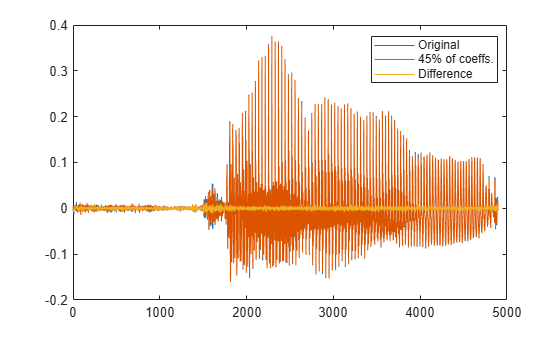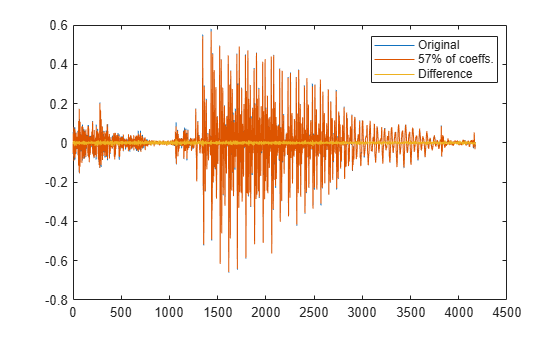# DCT for Speech Signal Compression

This example shows how to compress a speech signal using the discrete cosine transform (DCT).

Load a file containing the word "strong," spoken by a woman and by a man. The signals are sampled at 8 kHz.

```load('strong.mat') % To hear, type soundsc(her,fs), pause(1), soundsc(him,fs)```

Use the discrete cosine transform to compress the female voice signal. Decompose the signal into DCT basis vectors. There are as many terms in the decomposition as there are samples in the signal. The expansion coefficients in vector `X` measure how much energy is stored in each of the components. Sort the coefficients from largest to smallest.

```x = her'; X = dct(x); [XX,ind] = sort(abs(X),'descend');```

Find how many DCT coefficients represent 99.9% of the energy in the signal. Express the number as a percentage of the total.

```need = 1; while norm(X(ind(1:need)))/norm(X)<0.999 need = need+1; end xpc = need/length(X)*100;```

Set to zero the coefficients that contain the remaining 0.1% of the energy. Reconstruct the signal from the compressed representation. Plot the original signal, its reconstruction, and the difference between the two.

```X(ind(need+1:end)) = 0; xx = idct(X); plot([x;xx;x-xx]') legend('Original',[int2str(xpc) '% of coeffs.'],'Difference', ... 'Location','best')````% To hear, type soundsc(x,fs), pause(1), soundsc(xx,fs)`

Repeat the analysis for the male voice. Find how many DCT coefficients represent 99.9% of the energy and express the number as a percentage of the total.

```y = him'; Y = dct(y); [YY,ind] = sort(abs(Y),'descend'); need = 1; while norm(Y(ind(1:need)))/norm(Y)<0.999 need = need+1; end ypc = need/length(Y)*100;```

Set the rest of the coefficients to zero and reconstruct the signal from the compressed version. Plot the original signal, its reconstruction, and the difference between the two.

```Y(ind(need+1:end)) = 0; yy = idct(Y); plot([y;yy;y-yy]') legend('Original',[int2str(ypc) '% of coeffs.'],'Difference', ... 'Location','best')````% To hear, type soundsc(y,fs), pause(1), soundsc(yy,fs)`

In both cases, about half of the DCT coefficients suffice to reconstruct the speech signal reasonably. If the required energy fraction is 99%, the number of necessary coefficients reduces to about 20% of the total. The resulting reconstruction is inferior but still intelligible.

Analysis of these and other samples suggests that more coefficients are needed to characterize the man's voice than the woman's.